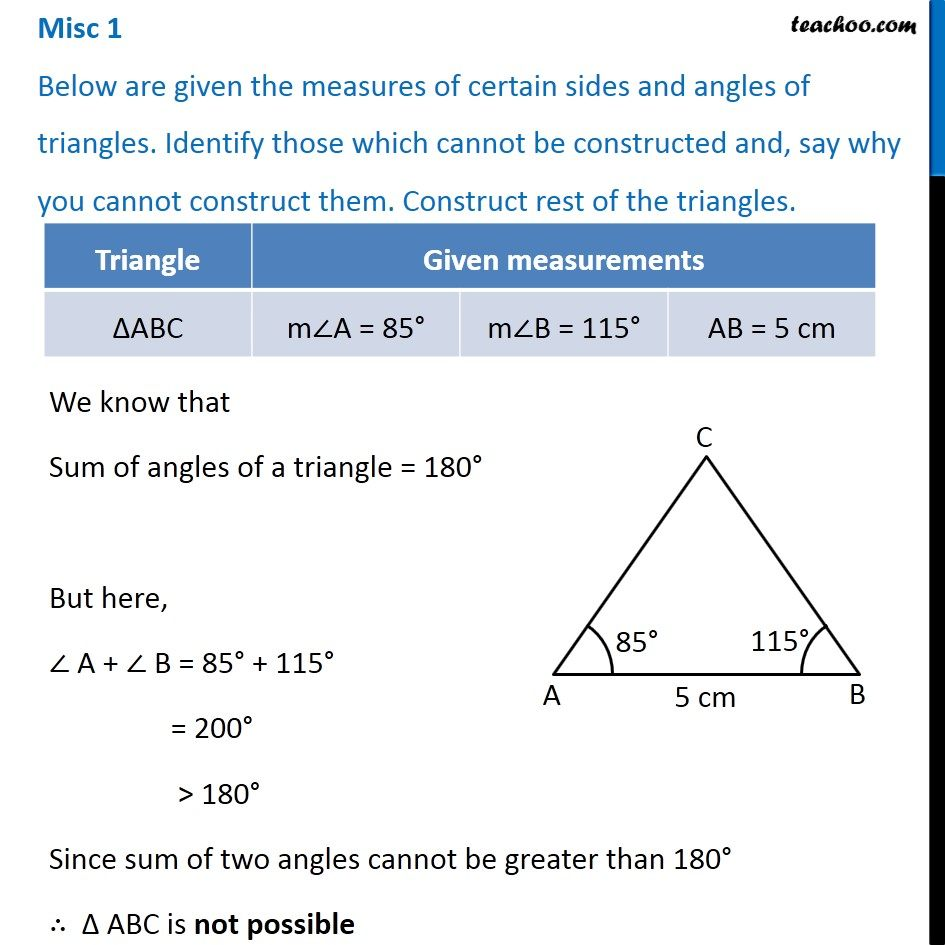1. Chapter 10 Class 7 Practical Geometry
2. Serial order wise
3. Miscellaneous

Transcript

Misc 1 Below are given the measures of certain sides and angles of triangles. Identify those which cannot be constructed and, say why you cannot construct them. Construct rest of the triangles. We know that Sum of angles of a triangle = 180° But here, ∠ A + ∠ B = 85° + 115° = 200° > 180° Since sum of two angles cannot be greater than 180° ∴ Δ ABC is not possible

Miscellaneous